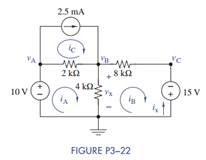Create an Account

Home / Questions / (a) Formulate mesh-current equations for the circuit in Figure P3−22. (b) Formulate...

(a) Formulate mesh-current equations for the circuit in Figure P3−22. (b) Formulate...

(a) Formulate mesh-current equations for the circuit in Figure P3−22.

(b) Formulate node-voltage equations for the circuit in Figure P3−22.

(c) Which set of equations would be easier to solve? Why?

(d) Find vx and ix using whichever method you prefer.Jul 28 2020 View more View LessSubscribe To Get Solution